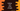# How to sort a list of tuple in python## How to sort a list of tuple in python:

In this post, we will learn how to sort a list of tuples in python.

We can sort a tuple similar to any other lists. But, we can also have tuples with multiple values. In that case, we can define which element to pick for the sorting.

In this post, I will show you how to sort a list of tuple using different ways.

### Example 1: Sort a simple list of tuple with two items for each:

Let’s try to sort a simple list of tuples with two items in each tuple. We can use list.sort() method to sort a list of tuples. For example:

``````given_list = [('a', 1), ('d', 4), ('b', 5), ('e', 2), ('c', 3)]

given_list.sort()
print(given_list)``````

Here,

• given_list is the given list of tuples, each holds two characters.
• We are using given_list.sort() to sort it.
• The last line is printing the sorted list, i.e. given_list.

If you run this program, it will print the below output:

``[('a', 1), ('b', 5), ('c', 3), ('d', 4), ('e', 2)]``

As you can see here, the tuples are sorted based on the first character on each.

We can also change the element that we want.

### Example 2: Sort using a different key:

We can define a key or the element that we want for the sorting. Suppose, we want to consider the second element for the sorting, not the first. We can change the above example as like below:

``````given_list = [('a', 1), ('d', 4), ('b', 5), ('e', 2), ('c', 3)]

given_list.sort(key=lambda x: x)
print(given_list)``````

If you run it, it will print the below output:

``[('a', 1), ('e', 2), ('c', 3), ('d', 4), ('b', 5)]``

As you can see now, the list is sorted based on the second element on each tuple.

### Example 3: Reverse sort:

sort method takes one key called reverse as the second parameter. If we pass True for it, it will sort the list in reverse order. So, let’s modify the above program as like below:

``````given_list = [('a', 1), ('d', 4), ('b', 5), ('e', 2), ('c', 3)]

given_list.sort(key=lambda x: x, reverse=True)
print(given_list)``````

If you run this code, it will print the below output:

``[('b', 5), ('d', 4), ('c', 3), ('e', 2), ('a', 1)]``

As you can see here, it is a reverse list considering the second key of the tuples.